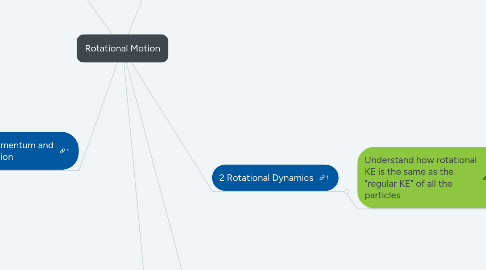# Rotational MotionJennifer Dick
Get Started. It's FreeRotational Motion## 1. 5 Angular Momentum and its Conservation

### 1.1. Compute angular momentum

1.1.1. Of moving particle about any axis

1.1.2. Of rigid body about fixed axis

1.1.3. Use the right-hand rule to specify the direction of:

1.1.3.1. Angular momentum vector for a moving particle

1.1.3.2. Angular velocity vector of rotating rigid object

1.1.3.3. Angular momentum vector for a rotating rigid object in simple cases where this vector lies in parallel to the angular velocity vector

### 1.2. Understand angular momentum conservation so they can:

1.2.1. State the relation between net external torque and angular momentum, and identify situations in which angular momentum is conserved

1.2.2. Analyze problems in which the moment of inertia of an object is changed as it rotates freely about a fixed axis

1.2.3. Analyze a collision between a moving particle and a rigid object that can rotate about a fixed axis or about its center of mass

## 2. 1 Rotational Kinematics

### 2.1. Relate the translational displacement, velocity, and acceleration of a point on a rigid object to its angular displacement, velocity, and acceleration about some fixed axis.

2.1.1. Understand the analogy between translational and rotational kinematics so they can write and apply equations relating the angular acceleration, angular velocity, and angular displacement of an object that rotates about a fixed axis with constant angular acceleration

2.1.2. Calculate and explain the difference between tangential acceleration, angular acceleration, and centripetal acceleration of a point on a rigid object.

## 3. 3 Torque

### 3.1. Calculate the magnitude of torque of a specified force about an arbitrary origin and specify the direction of rotation

3.1.1. Compute with vector cross-product

3.1.1.1. Use right-hand rule to specify direction of torque vector

3.1.2. Compute and understand torque from a geometric perspective

3.1.3. Calculate the torque on a rigid object due to gravity

3.1.4. Analyze dynamics of objects subject to a net torque

3.1.4.1. Set up appropriate coordinate system for rotational motion

3.1.4.2. Determine the angular acceleration with which a rigid object is accelerated about a fixed axis when subjected to a specified external torque or force

3.1.4.3. Analyze problems involving strings and massive pulleys

3.1.5. Analyze objects in static equilibrium

3.1.5.1. State the conditions for translational and rotational equilibrium of a rigid object

3.1.5.1.1. Apply these conditions in analyzing the equilibrium of a rigid object under the combined influence of a number of coplanar forces applied at different locations

## 4. 2 Rotational Dynamics

### 4.1. Understand how rotational KE is the same as the "regular KE" of all the particles

4.1.1. Compute kinetic energy of rigid body rotating about fixed axis

4.1.1.1. Moment of Inertia/Rotational Inertia

4.1.1.1.1. Develop qualitative understanding of rotational inertia

4.1.1.1.2. Develop skill in computing rotational inertia

## 5. 4 Rolling Motion

### 5.1. Understand the rolling motion of a rigid object along a surface

5.1.1. Calculate the total kinetic energy of an object that is undergoing both translational and rotational motion

5.1.1.1. Apply the equations of translational and rotational motion simultaneously in analyzing rolling without slipping

5.1.1.2. Apply conservation of energy to problems with rolling motion